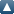# Items where Subject is "500 Scienze naturali e Matematica > 530 Fisica > 530.1 Teorie e fisica matematica"Up a level
 Export as ASCII CitationBibTeXDublin CoreEP3 XMLEndNoteGrid (abstract)HTML CitationJSONMETSMultiline CSVObject IDsOpenURL ContextObjectRDF+N-TriplesRDF+N3RDF+XMLReferReference Manager
Group by: Creators | Item Type
Number of items at this level: 11.

## B

Biller, Peter and Honerkamp, Joseph and Petruccione, Francesco (1991) Stochastic dynamical systems. Seminari di scienze, 10 . Istituto italiano per gli studi filosofici , Napoli. ISBN 8877230312

## C

Coppola, Fabrizio (1987) Le unità di controllo del supercalcolatore A.P.E. Masters thesis, Istituto Nazionale di Fisica Nuclare (INFN).

## N

Nardelli, Michele (2010) On the Lebesgue integral and the Lebesgue measure: mathematical applications in some sectors of Chern-Simons theory and Yang-Mills gauge theory and mathematical connections with some sectors of String Theory and Number Theory. Dip.Sc.Terra- Dip. Mat e Appl.. (Unpublished)

Nardelli, Michele A new possible Theory of Mathematical Connections between some Ramanujan's equations and Approximations to π, the equations of Inflationary Cosmology concerning the scalar field. Number Theory. (Unpublished)

Nardelli, Michele and Nardelli, Antonio (2021) Analyzing a formula concerning the Zeros of the Davenport Heilbronn Function. Further new possible mathematical connections with some equations concerning some sectors of String Theory and Supersymmetry Breaking. III. Theoretical Physics - Mathematics. (Unpublished)

Nardelli, Michele and Nardelli, Antonio From Ramanujan’s Mock Theta Functions to Black Hole Entropies and Particle Physics: Symmetry, Supersymmetry and Golden Ratio. Number Theory and Theoretical Physics. (Unpublished)

Nardelli, Michele and Nardelli, Antonio On the detailed analysis of the Table I of Ramanujan’s work “Modular equations and approximations to π". New possible mathematical connections with the Cosmological Constant values and some topics of String Theory. Theoretical Physics. (Unpublished)

Nardelli, Michele and Nardelli, Antonio On the study of various equations concerning the Isoperimetric Theorems. Possible mathematical connections with some sectors of Number Theory and Eternal Inflation model. Geometric Measure Theory and applications to the Theoretical Physics and Cosmology. (Unpublished)

Nardelli, Michele and Nardelli, Antonio On the study of various equations concerning the Isoperimetric Theorems. Possible mathematical connections with some sectors of Number Theory, String Theory and some cosmological parameters. Number Theory and String Theory. (Unpublished)

Nardelli, Michele and Nardelli, Antonio On the study of various Isoperimetric and Variational Problems. Possible mathematical connections with several parameters of Number Theory and sectors of String Theory. Number Theory, Geometric Measure Theory and Theoretical Physics. (Unpublished)

This list was generated on Fri Jun 9 19:12:07 2023 CEST.